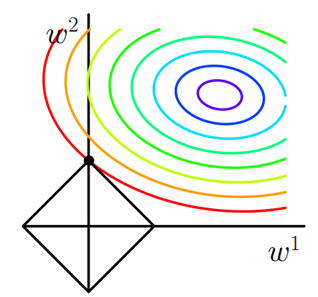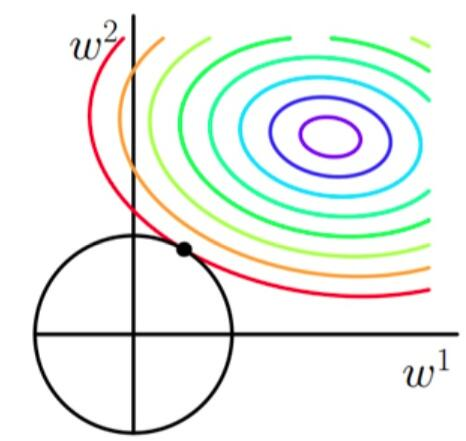# 损失函数 Loss function

## 均方误差 MSE

$$MSE = \frac{\sum\limits_{i=1}^n(y_i-\hat{y_i})^2}{n}$$

## 平均绝对值误差 MAE

$$MAE = \frac{\sum \limits_{i=1}^n \left| y_i-\hat{y_i}\right|}{n}$$

## R2 score

$R^2\ score$ 也称为决定系数, 越大越好, 解决了不同量纲下模型的效果好坏.
$$R^2 \ score = 1 - \frac{\sum \limits_{i=1}^n (y_i-\hat{y_i})^2}{\sum \limits_{i=1}^n (y_i-\bar{y_i})^2} = 1 - \frac{\sum \limits_{i=1}^n (y_i-\hat{y_i})^2 / n}{\sum \limits_{i=1}^n (y_i-\bar{y_i})^2/ n} = 1 - \frac{MSE}{Var}$$

## 交叉熵 Cross Entropy

### 二分类交叉熵

$$Entropy = -\sum^m_{i=1}\left[y_i\log{(1-p_i)} + (1-y_i)\log {p_i}\right]$$

### 多分类交叉熵

$$Entropy = -\sum^n_{i=1}\sum^m_{j=1}y_{ij}\log {p_{ij}}$$

## 正则化 Regularization

$$E_r(w; X, y)=E(w; X, y) + \alpha\Omega(w)$$

### L1正则化 L1 Regularization

$$\Omega(w)=\sum_i^W|w_i|$$

#### L1正则化为什么易于产生稀疏解?### L2正则化 L2 Regularization

$$\Omega(w)=\sum_i^Ww_i^2$$上一篇机器学习之模型选择

2020-08-02机器学习之评估指标

2020-07-31
目录